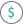#PPMT (PQL - xl)

This function returns the amount of payment of principal for a loan given the present value, specified interest rate, and number of terms

• Library: PQL \ Spreadsheet \ Financial
• Compatibility: Any content (regardless of data source) in the Tabulate spreadsheet module
• Solve: This function can be used with the Solve plug-in: both as part of the objective function and constraint functions

#### Syntax

PPMT(rate, per, nper, pv, OPTIONAL fv, OPTIONAL type)

##### Function Arguments
 Name Description Type Optional rate Value of interest rate per period Number per Number of the period for which to find the interest, between 1 and nper Number nper Total number of payment periods in an annuity Number pv Present value, worth now Number fv Future value, cash value after the last payment; if omitted, the calculation uses zero Number Y type Indicates when payments are due; at the end (0) or beginning (1) of the period; if omitted, the calculation uses the end (0) Number Y